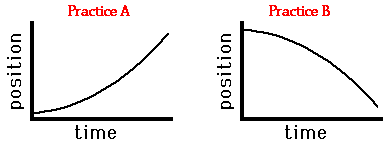# Period 5 Kinematics Review

18 Questions | Attempts: 71
ShareSettings• 1.
Which of the following is a distance?
• A.

2.65 m east

• B.

9.8 m down

• C.

11 m

• D.

-3.4 m

• 2.
Which of the following is a velocity?
• A.

3.5 m/s

• B.

.04 m/s

• C.

-5.0 m/s

• D.

-9.8 m/s/s

• 3.
What is the acceleration due gravity?
• A.

-9.8 m/s/s

• B.

-9.8 m/s

• C.

+9.8 m/s

• D.

+9.8 m/s/s

• 4.
You drive 100 mi North and then 20 mi south. What is your distance?
• A.

80 mi North

• B.

80 mi

• C.

120 mi North

• D.

120 mi

• 5.
You drive 100 mi North. You then drive 20 miles south. What was your displacement?
• A.

80 m North

• B.

80 m South

• C.

120 m

• D.

120 m North

• 6.
Chose all the the scenarios where you are accelerating:
• A.

You are standing still, watching your crush walk away from you.

• B.

You are running away at a constant velocity.

• C.

You are rounding a corner going a constant 20 m/s.

• D.

You see a beautiful, new, smiling stranger, who makes you slow to a stop.

• 7.
Choose the situations where the object is speeding up:
• A.

Positive velocity, positive acceleration

• B.

Positive velocity, negative acceleation

• C.

Negative velocity, positive acceleration

• D.

Negative velocity, negative acceleration

• 8.
Choose the situations where the object is slowing down:
• A.

Positive velocity, positive acceleration

• B.

Positive velocity, negative acceleation

• C.

Negative velocity, positive acceleration

• D.

Negative velocity, negative acceleration

• 9.
You stand at the top of the highest building in the world, the Burj Kalifa, which is about 818 m tall. Imagine there is no air to get in the way. You drop a penny. How long would it take the penny to hit the ground?
• A.

166.9 s

• B.

41.7 s

• C.

12.9 s

• D.

20.4 s

• 10.
The object in the graph on the left is:
• A.

Speeding up in the positive direction

• B.

Slowing down in the positive direction

• C.

Speeding up in the negative direction

• D.

Slowing down in the negative direction

• 11.
The object in the graph on the right
• A.

Speeding up in the positive direction

• B.

Slowing down in the positive direction

• C.

Speeding up in the negative direction

• D.

Slowing down in the negative direction

• 12.
Describe the motion of the object in this graph.
• A.

It is stopped, then moving at a steady constant velocity.

• B.

It is moving at a constant velocity, then going a faster constant velocity

• C.

If is moving at a constant velocity, then accelerating.

• D.

It is stopped, then slowing down.

• 13.
During the first 4 seconds, what is the acceleration of the object?
• A.

2 m/s/s

• B.

2 m/s

• C.

0 m/s/s

• D.

• 14.
What was the total displacement of the object in this graph?
• A.

48 m

• B.

2 m

• C.

24 m

• D.

• 15.
Match the velocity graph to its acceleration graph.
• A.

A

• B.

B

• C.

C

• D.

D

• 16.
A zebra is being chased by a lion. It is running constantly at its top velocity +22 m/s. How long will it take the zebra to run 68 m?
• A.

46

• B.

0.32 s

• C.

1496 s

• D.

3.1 s

• 17.
A plane goes from flying 220 m/s to stop while experiancing an acceleration of -16 m/s/s. How far does the plane travel before it comes to a stop?
• A.

13.75 m

• B.

24200 m

• C.

27.5

• D.

1512.5 m

• 18.
During the last 4 seconds, what is the acceleration of the object?
• A.

2 m/s/s

• B.

2 m/s

• C.

0 m/s/s

• D.Back to top